# 4. Unidimensional Numeric Data and Their Empirical Distribution

The online version of the open-access textbook Minimalist Data Wrangling with Python by Marek Gagolewski is, and will remain, freely available for everyone’s enjoyment (also in PDF). Any bug/typos reports/fixes are appreciated. Although available online, this is a whole course; it should be read from the beginning to the end. In particular, refer to the Preface for general introductory remarks.

Our data wrangling adventure starts the moment we get access to, or decide to collect, dozens of data points representing some measurements, such as sensor readings for some industrial processes, body measures for patients in a clinic, salaries of employees, sizes of cities, etc.

For instance, consider the heights of adult females (>= 18 years old, in cm) in the longitudinal study called National Health and Nutrition Examination Survey (NHANES) conducted by the US Centres for Disease Control and Prevention.

heights = np.loadtxt("https://raw.githubusercontent.com/gagolews/" +


Let us preview a few observations:

heights[:6]  # first six
## array([160.2, 152.7, 161.2, 157.4, 154.6, 144.7])


This is an example of quantitative (numeric) data. They are in the form of a series of numbers. It makes sense to apply various mathematical operations on them, including subtraction, division, taking logarithms, comparing which one is greater than the other, and so forth.

Most importantly, here, all the observations are independent of each other. Each value represents a different person Our data sample consists of 4221 points on the real line (a bag of points whose actual ordering of does not matter). From Figure 4.1, we see that merely looking at the numbers themselves tells us nothing. There are too many of them.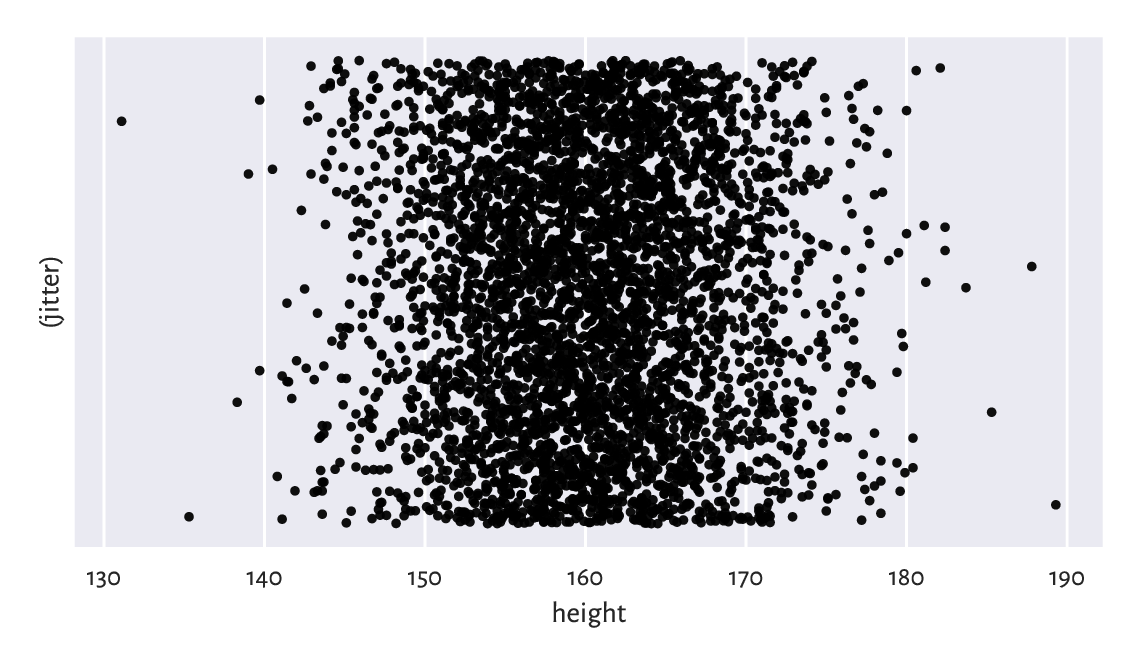Figure 4.1 The heights dataset is comprised of independent points on the real line. We have added some jitter on the y-axis for dramatic effects only: the points are too plentiful

This is why we are interested in studying a multitude of methods that can bring some insight into the reality behind the numbers. For example, inspecting their distribution.

## 4.1. Creating Vectors in numpy

In this chapter we introduce basic ways to create numpy vectors, which are an efficient data structure for storing and operating on numeric data just like the ones above.

numpy [H+20] is an open-source add-on for numerical computing written by Travis Oliphant and other developers in 2005 (although the project has a much longer history and stands on the shoulders of many giants (e.g., concepts from the APL and Fortran languages). It adds support for multi-dimensional arrays and numerous operations on them, similar to those available in R, S, GNU Octave, Scilab, Julia, Perl (via Perl Data Language), and some numerical analysis libraries such as LAPACK, GNU GSL, etc.

Many other packages are built on top of numpy (including scipy [V+20], pandas [McK17], and sklearn [PVG+11]), hence our studying it in very detail is utterly important. Whatever we learn about vectors will be greatly transferable to the case of the processing of data frame columns.

It is customary to import the numpy package under the np alias:

import numpy as np


Hence, our code can now refer to the objects defined therein as np.spam, np.bacon, or np.spam.

### 4.1.1. Enumerating Elements

One way to create a vector is by calling the numpy.array function:

x = np.array([10, 20, 30, 40, 50, 60])
x
## array([10, 20, 30, 40, 50, 60])


Here, the vector elements were specified by means of an ordinary list. Ranges and tuples can also be used as content providers, which the kind reader is encouraged to check themself.

A vector of length (size) n is often used to represent a point in an n dimensional space (for example, GPS coordinates of a place on Earth assume n=2) or n readings of some 1-dimensional quantity (e.g., recorded heights of n people).

The said length can either be read using the previously mentioned len function:

len(x)
## 6


or by reading the array’s shape slot:

x.shape
## (6,)


A vector is a 1-dimensional array, hence its shape is stored as a tuple of length 1 (the number of dimensions is given by querying x.ndim). We can therefore get its length by accessing x.shape.

On a side note, matrices (2-dimensional arrays), which we shall study in Chapter 7, will be of shape like (number_of_rows, number_of_columns).

Recall that Python lists, e.g., [1, 2, 3], represent simple sequences of objects of any kind. Their use cases are very broad, which is both an advantage and something quite the opposite. Vectors in numpy are like lists, but on steroids. They are powerful in scientific computing because of the underlying assumption that each object they store is of the same type1. Although it is possible to save references to arbitrary objects therein, in most scenarios we will be dealing with vectors of logical values, integers, and floating-point numbers. Thanks to this, a wide range of applicable methods could have been defined to enable the performing of the most popular mathematical operations.

And so, above we have created a sequence of integers:

x.dtype  # data type
## dtype('int64')


However, other element types are possible too. For instance, we can convert the above to a float vector:

x.astype(float)  # or np.array(x, dtype=float)
## array([10., 20., 30., 40., 50., 60.])


Let us emphasise that the above is now printed differently (compare the output of print(x) above).

Furthermore:

np.array([True, False, False, True])
## array([ True, False, False,  True])


gave a logical vector; the constructor detected that the common type of all the elements is bool. Also:

np.array(["spam", "spam", "bacon", "spam"])
## array(['spam', 'spam', 'bacon', 'spam'], dtype='<U5')


This yielded an an array of strings in Unicode (i.e., capable of storing any character in any alphabet, emojis, mathematical symbols, etc.), each of no more than 5 code points in length. It is important to know this, because, as we will point out in Chapter 14, replacing any element with new content will result in the too long strings’ truncation. This can be remedied by calling x.astype("<U10"), for example.

### 4.1.2. Arithmetic Progressions

numpy’s arange is similar to the built-in range, but outputs a vector:

np.arange(0, 10, 2)  # from 0 to 10 (exclusive) by 2
## array([0, 2, 4, 6, 8])


numpy.linspace (linear space) creates a sequence of equidistant points in a given interval:

np.linspace(0, 1, 5)  # from 0 to 1 (inclusive), 5 equispaced values
## array([0.  , 0.25, 0.5 , 0.75, 1.  ])

Exercise 4.1

Call help(np.linspace) or help("numpy.linspace") to study the meaning of the endpoint argument. Find the same documentation page at the numpy project’s website. Another way is to use your favourite search engine such as DuckDuckGo and query “linspace site:numpy.org2. Always remember to gather information from first-hand sources. You should become a frequent visitor of this page (and similar ones). In particular, every so often it is a good idea to check out for significant updates at https://numpy.org/news/.

### 4.1.3. Repeating Values

numpy.repeat repeats each value a given number of times:

np.repeat(5, 6)
## array([5, 5, 5, 5, 5, 5])
np.repeat([1, 2], 3)
## array([1, 1, 1, 2, 2, 2])
np.repeat([1, 2], [3, 5])
## array([1, 1, 1, 2, 2, 2, 2, 2])


In each case, every element from the list passed as the 1st argument was repeated the corresponding number of times, as defined by the 2nd argument. The kind reader should not expect us to elaborate upon the obtained results any further, because everything is evident: they need to look at the example calls, carefully study all the displayed outputs, and make the conclusions by themself. If something is unclear, they should consult the official documentation and apply Rule #4.

Moving on. numpy.tile, on the other hand, repeats a whole sequence with recycling:

np.tile([1, 2], 3)
## array([1, 2, 1, 2, 1, 2])


Notice the difference between the above and the result of numpy.repeat([1, 2], 3).

See also3 numpy.zeros and numpy.ones for some specialised versions of the above.

### 4.1.4. numpy.r_ (*)

numpy.r_ gives perhaps the most flexible means for creating vectors involving quite a few of the aforementioned scenarios, however it has a quirky syntax.

For example:

np.r_[1, 2, 3, np.nan, 5, np.inf]
## array([ 1.,  2.,  3., nan,  5., inf])


Here, nan stands for a not-a-number and is used as a placeholder for missing values (discussed in Section 15.1) or wrong results, such as the square root of -1 in the domain of reals. The inf object, on the other hand, means infinity, $$\infty$$. We can think of it as value that is too large to be represented in the set of floating-point numbers.

We see that numpy.r_ uses square brackets instead of the round ones. This is actually smart, because we have mentioned in Section 3.2.2 that slices (:) cannot be used outside them. And so:

np.r_[0:10:2]  # like np.arange(0, 10, 2)
## array([0, 2, 4, 6, 8])


What is more, it accepts the following syntactic sugar:

np.r_[0:1:5j]  # like np.linspace(0, 1, 5)
## array([0.  , 0.25, 0.5 , 0.75, 1.  ])


Here, 5j does not have the literal meaning (a complex number). By an arbitrary convention, and only in this context, it denotes the output length of the sequence to be generated. Could the numpy authors do that? Well, they could, and they did. End of story.

Finally, we can combine many chunks into one:

np.r_[1, 2, *2, 0:3, 0:3:3j]
## array([1. , 2. , 3. , 3. , 0. , 1. , 2. , 0. , 1.5, 3. ])


### 4.1.5. Generating Pseudorandom Variates

The automatically attached numpy.random module defines many functions to generate pseudorandom numbers. We will be discussing the reason for our using the pseudo prefix in Section 6.4, so now let us only quickly take note of a way to sample from the uniform distribution on the unit interval:

np.random.rand(5)  # 5 pseudorandom observations in [0, 1]
## array([0.49340194, 0.41614605, 0.69780667, 0.45278338, 0.84061215])


and to pick a few values from a given set with replacement (so that any number can be generated multiple times):

np.random.choice(np.arange(1, 10), 20)  # replace=True
## array([7, 7, 4, 6, 6, 2, 1, 7, 2, 1, 8, 9, 5, 5, 9, 8, 1, 2, 6, 6])


We will usually be reading whole heterogeneous tabular data sets using pandas.read_csv, being the topic we shall cover in Chapter 10.

It is worth knowing, though, that arrays with elements of the same type can be read efficiently from text (e.g., CSV) files using numpy.loadtxt. See the code chunk at the beginning of this chapter for an example.

Exercise 4.2

## 4.2. Mathematical Notation

Mathematically, we will be denoting number sequences with:

$\boldsymbol{x}=(x_1, x_2, \dots, x_n),$

where $$x_i$$ is the $$i$$-th element therein and $$n$$ is the length (size) of the tuple. Using the programming syntax, $$n$$ corresponds to len(x) or, equivalently, x.shape. Furthermore, $$x_i$$ is x[i-1] (because the first element is at index 0).

The bold font (hopefully visible) is to emphasise that $$\boldsymbol{x}$$ is not an atomic entity ($$x$$), but rather a collection thereof. For brevity, instead of saying “let $$\boldsymbol{x}$$ be a real-valued sequence4 of length $$n$$”, we shall write “let $$\boldsymbol{x}\in\mathbb{R}^n$$”. Here:

• the “$$\in$$” symbol stands for “is in” or “is a member of”,

• $$\mathbb{R}$$ denotes the set of real numbers (the very one that features, $$0$$, $$-358745.2394$$, $$42$$ and $$\pi$$, amongst uncountably many others), and

• $$\mathbb{R}^n$$ is the set of real-valued sequences of length $$n$$ (i.e., $$n$$ such numbers considered at a time); e.g., $$\mathbb{R}^2$$ includes pairs such as $$(1, 2)$$, $$(\pi/3, \sqrt{2}/2)$$, and $$(1/3, 10^{3})$$.

Note

Mathematical notation is pleasantly abstract (general) in the sense that $$\boldsymbol{x}$$ can be anything, e.g., data on the incomes of households, sizes of the largest cities in some country, or heights of participants in some longitudinal study. At a first glance, such a representation of objects from the so-called real world might seem overly simplistic, especially if we wish to store information on very complex entities. However, in most cases, expressing them as vectors (i.e., establishing a set of numeric attributes that best describe them in a task at hand) is not only natural but also perfectly sufficient for achieving whatever we aim at.

Exercise 4.3

Consider the following problems:

• How would you represent a patient in a clinic (for the purpose of conducting research in cardiology)?

• How would you represent a car in an insurance company’s database (for the purpose of determining how much a driver should pay annually for the mandatory policy)?

• How would you represent a student in a university (for the purpose of granting them scholarships)?

In each case, list a few numeric features that best describe the reality of concern. On a side note, descriptive (categorical) labels can always be encoded as numbers, e.g., female = 1, male = 2, but this will be the topic of Chapter 11.

Furthermore, by $$x_{(i)}$$ (notice the bracket5) we will denote the i-th smallest value in $$\boldsymbol{x}$$ (also called the $$i$$-th order statistic). In particular, $$x_{(1)}$$ is the sample minimum and $$x_{(n)}$$ is the maximum. The same in Python:

x = np.array([5, 4, 2, 1, 3])  # just an example
x_sorted = np.sort(x)
x_sorted, x_sorted[-1]  # the minimum and the maximum
## (1, 5)


Also, to avoid the clutter of notation, in certain formulae (e.g., in the definition of the type-7 quantiles in Section 5.1.1.3), we will be assuming that $$x_{(0)}$$ is the same as $$x_{(1)}$$ and $$x_{(n+1)}$$ is equivalent to $$x_{(n)}$$.

## 4.3. Inspecting the Data Distribution with Histograms

Histograms are one of the most intuitive tools for depicting the empirical distribution of a data sample. We will be drawing them using the statistical data visualisation package called seaborn [Was21] (written by Michael Waskom), which was built on top of the classic plotting library matplotlib [Hun07] (originally developed by John D. Hunter). Let us import both packages and set their traditional aliases:

import matplotlib.pyplot as plt
import seaborn as sns


Note

It is customary to call a single function from seaborn and then perform a series of additional calls to matplotlib to tweak the display details. It is important to remember that the former uses the latter to achieve its goals, not the other way around. In many exercises, seaborn might not even have the required functionality at all, and we will be using matplotlib only, and nothing else.

### 4.3.1. heights: A Bell-Shaped Distribution

Let us draw a histogram of the heights dataset, see Figure 4.2.

sns.histplot(heights, bins=11, color="lightgray")
plt.show()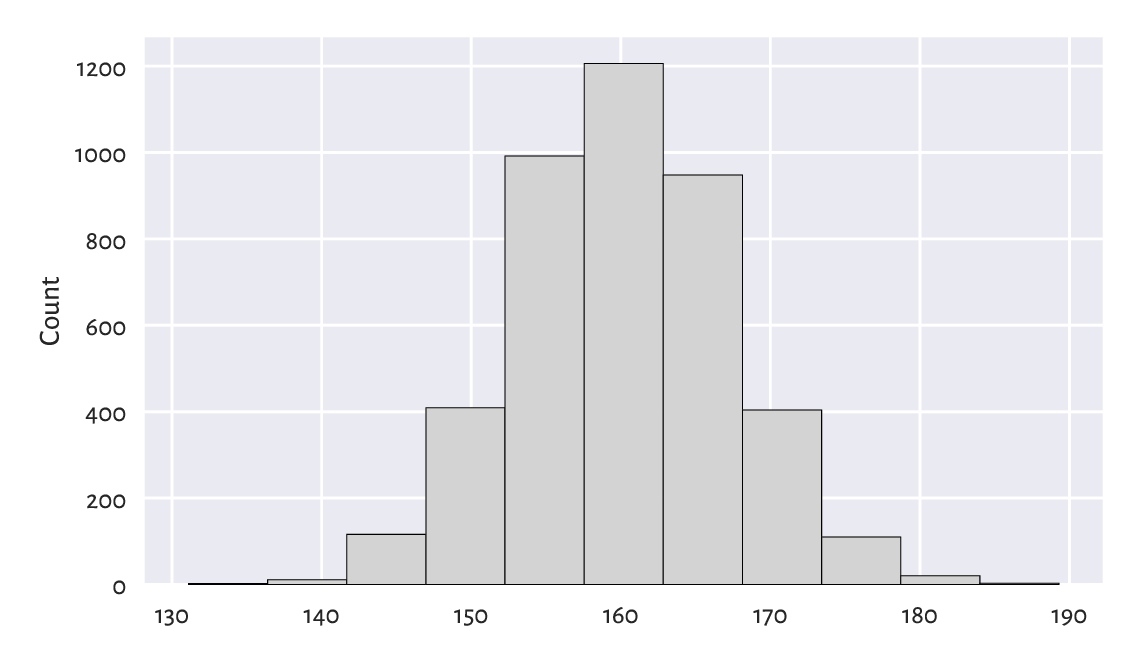Figure 4.2 Histogram of the heights dataset; the empirical distribution is nicely bell-shaped

The data were split into 11 bins and plotted in such a way that the bar heights are proportional to the number of observations falling into each interval. The bins are non-overlapping, adjacent to each other, and of equal lengths. We can read their coordinates by looking at the bottom side of each rectangular bar. For example, there are ca. 1200 observations falling into the interval $$[158, 163]$$ (more or less) and ca. 400 in $$[168, 173]$$ (approximately).

This distribution is bell-shaped6 – nicely symmetrical around about 160 cm. The most typical (normal) observations are somewhere in the middle, and the probability mass decreases quickly on both sides. Actually, in Chapter 6 we will model this dataset using a normal distribution and obtain an excellent fit. In particular, we will mention that observations outside the interval $$[139, 181]$$ are very rare (probability less than 1%; via the 3σ rule, i.e., expected value ± 3 standard deviations).

### 4.3.2. income: A Right-Skewed Distribution

For some of us, a normal distribution is a prototypical one – we might expect (wishfully think) that many phenomena yield similar regularities. And that is indeed the case7, e.g., in psychology (IQ or personality tests), physiology (the above heights), or when measuring stuff with not-so-precise devices (distribution of errors). We might be tempted to think now that everything is normally distributed, but this is very much untrue.

Let us therefore consider another dataset. In Figure 4.3 we depict the distribution of a simulated sample of disposable income of 1000 randomly chosen UK households, in British Pounds, for the financial year ending 2020 (unfortunately, the UK Office for National Statistics does not provide us with details on each taxpayer, for privacy and other reasons, hence we needed to recreate it based on data from this report):

income = np.loadtxt("https://raw.githubusercontent.com/gagolews/" +
"teaching_data/master/marek/uk_income_simulated_2020.txt")
sns.histplot(income, stat="percent", bins=20, color="lightgray")
plt.show()Figure 4.3 Histogram of the income dataset; the distribution is right-skewed

We have normalised (stat="percent") the bar heights so that they all sum to 1 (or, equivalently, 100%), which resulted in a probability histogram.

Now we see that the probability density quickly increases, reaches its peak at around £15,500–£35,000 and then slowly goes down. We say that it has a long tail on the right (and hence it is right- or positive-skewed), which means that high or ever very high salaries are still quite likely. Thus, it is quite a non-normal distribution. Most people are rather poor, they do not earn the average salary. We will get back to that later.

Note

Looking at Figure 4.3, we might have taken note of the relatively higher bars, as compared to their neighbours, at ca. £100,000 and £120,000. We might be tempted to try to invent a story about why there can be some difference in the relative probability mass, but we should refrain from it. Our data sample is quite small, and it is likely that they are merely due to some natural variability (Section 6.4.4). Of course, there might be some reasons behind it (theoretically), but we cannot read this only by looking at a single histogram. In other words, it is a tool that we use to identify some rather general features of the data distribution (like the overall shape), not the specifics.

Exercise 4.4

There is also the nhanes_adult_female_weight_2020 dataset in our data repository, giving corresponding weights (in kilograms) of the NHANES study participants. Draw a histogram. Does its shape resemble the income or heights distribution more?

### 4.3.3. How Many Bins?

Unless some stronger assumptions about the data distribution are made, choosing the right number of bins is more art than science:

• too many will result in a rugged histogram,

• too few might result in our missing of important details.

Figure 4.4 illustrates this.

plt.subplot(1, 2, 1)  # 1 row, 2 columns, 1st plot
sns.histplot(income, bins=5, color="lightgray")
plt.subplot(1, 2, 2)  # 1 row, 2 columns, 2nd plot
sns.histplot(income, bins=200, color="lightgray")
plt.ylabel(None)
plt.show()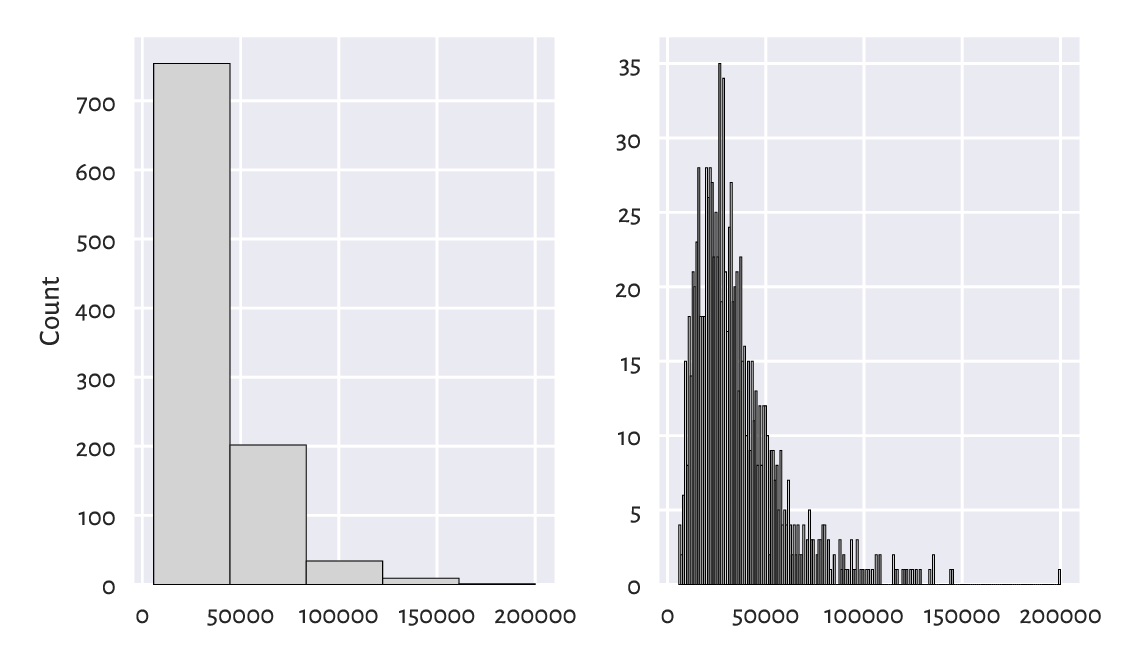Figure 4.4 Too few and too many histogram bins (the income dataset)

For example, in the histogram with 5 bins, we miss the information that the ca. £20,000 income is more popular than the ca. £10,000 one. (as given by the first two bars in Figure 4.3).

On the other hand, the histogram with 200 bins already seems too fine-grained.

Important

Usually, the “truth” is probably somewhere in-between. When preparing histograms for publication (e.g., in a report or on a webpage), we might be tempted to think “one must choose one and only one bin count”. In fact, we do not have to – even though some people will insist on it, remember that it is we who are responsible for the data be presented in the most unambiguous fashion possible. Thus providing 2 or 3 histograms can sometimes be a much better idea.

Further, let us be aware that someone might want to trick us by choosing the number of bins that depict the reality in good light, when the truth is quite the opposite. For instance, the histogram on the left above hides the poorest households inside the first bar – the first income bracket is very wide. If we cannot request access to the original data, best thing we can do is to simply ignore such a data visualisation instance and warn others not to trust it. A true data scientist must be a sceptic.

The documentation of seaborn.histplot (and the underlying matplotlib.pyplot.hist), states that the bins argument is passed to numpy.histogram_bin_edges to determine the intervals into which our data are to be split. numpy.histogram uses the same function and additionally returns the corresponding counts (how many observations fall into each bin) instead of plotting them.

counts, bins = np.histogram(income, 20)
counts
## array([131, 238, 238, 147,  95,  55,  29,  23,  10,  12,   5,   7,   4,
##          3,   2,   0,   0,   0,   0,   1])
bins
## array([  5750.  ,  15460.95,  25171.9 ,  34882.85,  44593.8 ,  54304.75,
##         64015.7 ,  73726.65,  83437.6 ,  93148.55, 102859.5 , 112570.45,
##        122281.4 , 131992.35, 141703.3 , 151414.25, 161125.2 , 170836.15,
##        180547.1 , 190258.05, 199969.  ])


Thus, there are 238 observations both in the $$[15{,}461; 25{,}172)$$ and $$[25{,}172; 34{,}883)$$ intervals.

Note

A table of ranges and the corresponding counts can be useful for data reporting. It is more informative and takes less space than a series of raw numbers, especially if we present them like in the table below.

Table 4.1 Incomes of selected British households; the bin edges are pleasantly round numbers

income bracket [£1000s]

count

0-200

236

200-400

459

400-600

191

600-800

64

800-1000

26

1000-1200

11

1200-1400

10

1400-1600

2

1600-1800

0

1800-2000

1

Reporting data in tabular form can also increase privacy of the subjects (making subjects less identifiable, which is good) or hide some uncomfortable facts (which is not so good; “there are 10 people in our company earning more than £200,000 p.a.” – this can be as much as £10,000,000, but shush).

Exercise 4.5

Find how we can provide the seaborn.histplot and numpy.histogram functions with custom bin breaks. Plot a histogram where the bin edges are 0, 20,000, 40,000, etc. (just like in the above table). Also let us highlight the fact that bins do not have to be of equal sizes: set the last bin to $$[140{,}000; 200{,}000]$$.

Example 4.6

Let us also inspect the bin edges and counts that we see in Figure 4.2:

counts, bins = np.histogram(heights, 11)
counts
## array([   2,   11,  116,  409,  992, 1206,  948,  404,  110,   20,    3])
bins
## array([131.1       , 136.39090909, 141.68181818, 146.97272727,
##        152.26363636, 157.55454545, 162.84545455, 168.13636364,
##        173.42727273, 178.71818182, 184.00909091, 189.3       ])

Exercise 4.7

(*) There are quite a few heuristics to determine the number of bins automagically, see numpy.histogram_bin_edges for a few formulae. Check out how different values of the bins argument (e.g., "sturges", "fd") affect the histogram shapes on both income and heights datasets. Each has its own limitations, none is perfect, but some might be a good starting point for further fine-tuning.

We will get back to the topic of manual data binning in Section 11.1.5.

### 4.3.4. peds: A Bimodal Distribution (Already Binned)

Sometimes data we get access to have already been binned by somebody else. For instance, here are the December 2021 hourly average pedestrian counts near the Southern Cross Station in Melbourne:

peds = np.loadtxt("https://raw.githubusercontent.com/gagolews/" +
"teaching_data/master/marek/southern_cross_station_peds_2019_dec.txt")
peds
## array([  31.22580645,   18.38709677,   11.77419355,    8.48387097,
##           8.58064516,   58.70967742,  332.93548387, 1121.96774194,
##        2061.87096774, 1253.41935484,  531.64516129,  502.35483871,
##         899.06451613,  775.        ,  614.87096774,  825.06451613,
##        1542.74193548, 1870.48387097,  884.38709677,  345.83870968,
##         203.48387097,  150.4516129 ,  135.67741935,   94.03225806])


We cannot thus use seaborn.histplot to depict them. Instead, we can rely on a more low-level function, matplotlib.pyplot.bar, see Figure 4.5.

plt.bar(np.arange(0, 24), width=1, height=peds,
color="lightgray", edgecolor="black", alpha=0.8)
plt.show()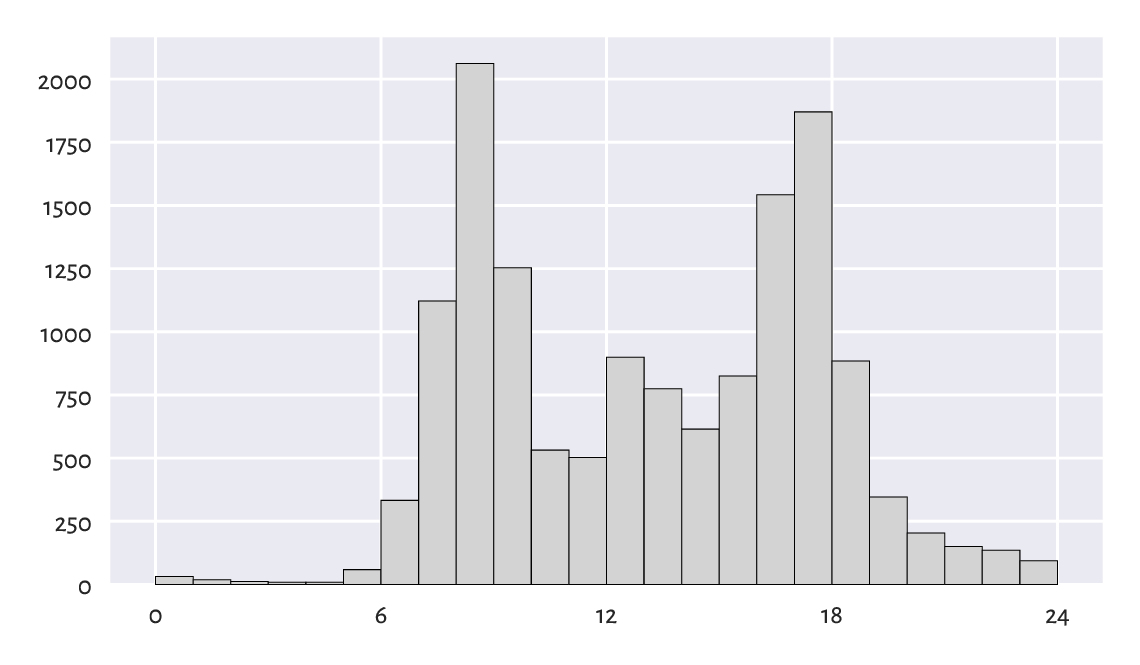Figure 4.5 Histogram of the peds dataset; a bimodal (trimodal?) distribution

This is an example of a bimodal (or even trimodal) distribution: there is a morning peak and an evening peak (and some analysts probably would distinguish a lunchtime one too).

### 4.3.5. matura: A Bell-Shaped Distribution (Almost)

Figure 4.6 depicts a histogram of another interesting dataset which comes in an already pre-summarised form.

matura = np.loadtxt("https://raw.githubusercontent.com/gagolews/" +
"teaching_data/master/marek/matura_2019_polish.txt")
plt.bar(np.arange(0, 71), width=1, height=matura,
color="lightgray", edgecolor="black", alpha=0.8)
plt.show()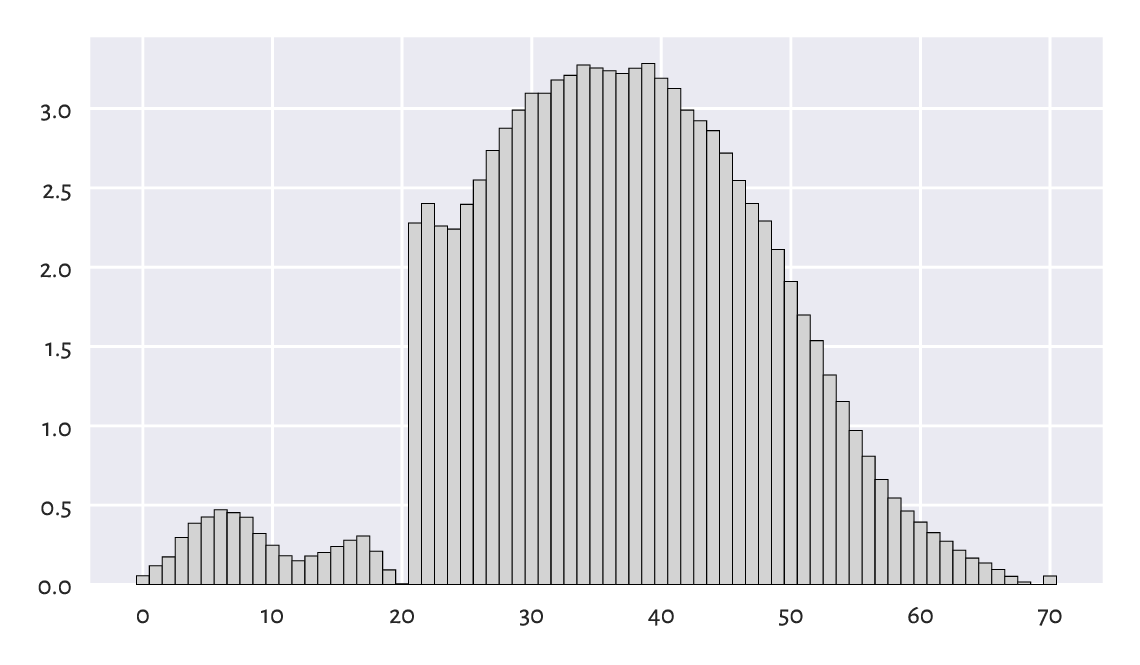Figure 4.6 Histogram of matura dataset; a bell-shaped distribution… almost

This gives the distribution of the 2019 Matura (end of high school) exam scores in Poland (in %) – Polish literature8 at basic level.

It seems that the distribution should be bell-shaped, but someone tinkered with it. However, knowing that:

• the examiners are good people – we teachers love our students,

• 20 points were required to pass,

• 50 points were given for an essay – and beauty is in the eye of beholder,

this actually starts to make sense. Without graphically depicting this dataset, we would not know that such (albeit lucky for some students) anomalies occurred.

### 4.3.6. marathon (Truncated – Fastest Runners): A Left-Skewed Distribution

Next, let us consider the 37th PZU Warsaw Marathon (2015) results.

marathon = np.loadtxt("https://raw.githubusercontent.com/gagolews/" +
"teaching_data/master/marek/37_pzu_warsaw_marathon_mins.txt")


Here are the top 5 gun times (in minutes):

marathon[:5]  # preview first 5 (data are already sorted increasingly)
## array([129.32, 130.75, 130.97, 134.17, 134.68])


Plotting the histogram of the data on the participants who finished the 42.2 km run in less than three hours, i.e., a truncated version of this dataset, reveals that the data are highly left-skewed, see Figure 4.7.

sns.histplot(marathon[marathon < 180], color="lightgray")
plt.show()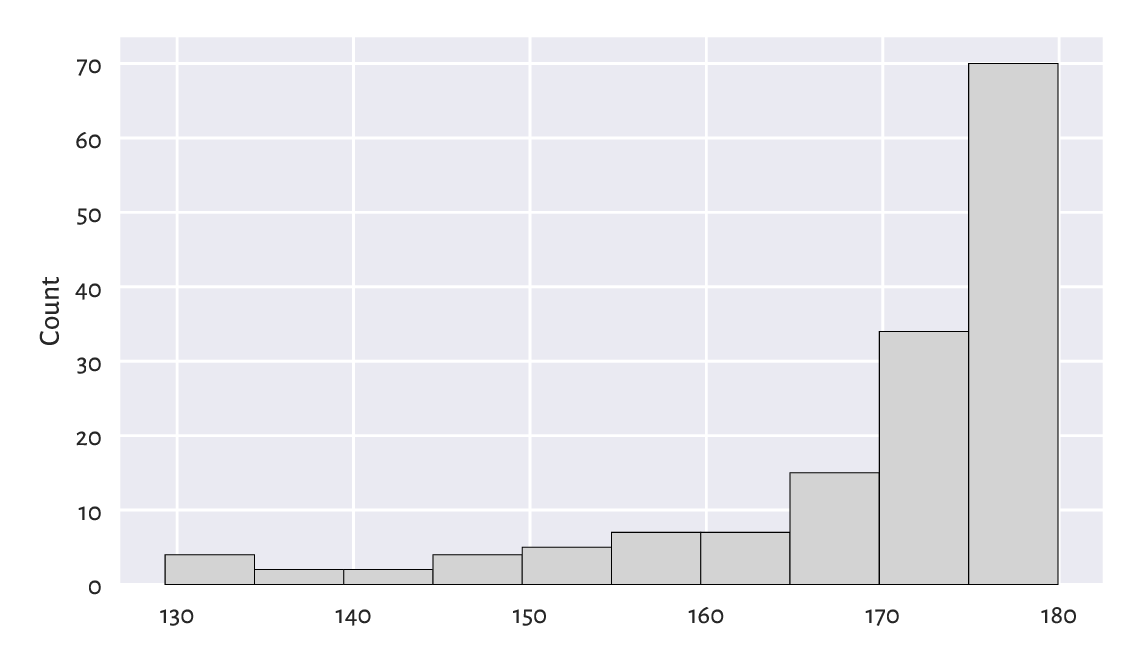Figure 4.7 Histogram of a truncated version of the marathon dataset; the distribution is left-skewed

This was of course expected – there are only few elite runners in the game. Yours truly wishes his personal best becomes < 180 minutes someday. We shall see. Running is fun, so is walking; why not taking a break for an hour and going outside?

Exercise 4.8

Plot the histogram of the untruncated (complete) version of this dataset.

### 4.3.7. Log-scale and Heavy-Tailed Distributions

Consider the dataset on the populations of cities in the 2000 US Census:

cities = np.loadtxt("https://raw.githubusercontent.com/gagolews/" +
"teaching_data/master/other/us_cities_2000.txt")


Let us restrict ourselves only to the cities whose population is not less than 10,000 (another instance of truncating, this time on the other side of the distribution). It turns out that, even though they constitute ca. 14% of all the US settlements, as much as about 84% of all the citizens live there.

large_cities = cities[cities >= 10000]


Here are the populations of the 5 largest cities (can we guess which ones are they?):

large_cities[-5:]  # preview last 5 – data are sorted increasingly
## array([1517550., 1953633., 2896047., 3694742., 8008654.])


The histogram is depicted in Figure 4.8. It is virtually unreadable, because the distribution is not just right-skewed; it is extremely heavy-tailed: most cities are small, and those that are large – such as New York – are really unique. Had we plotted the whole dataset (cities instead of large_cities), the results’ intelligibility would be even worse.

sns.histplot(large_cities, bins=20, color="lightgray")
plt.show()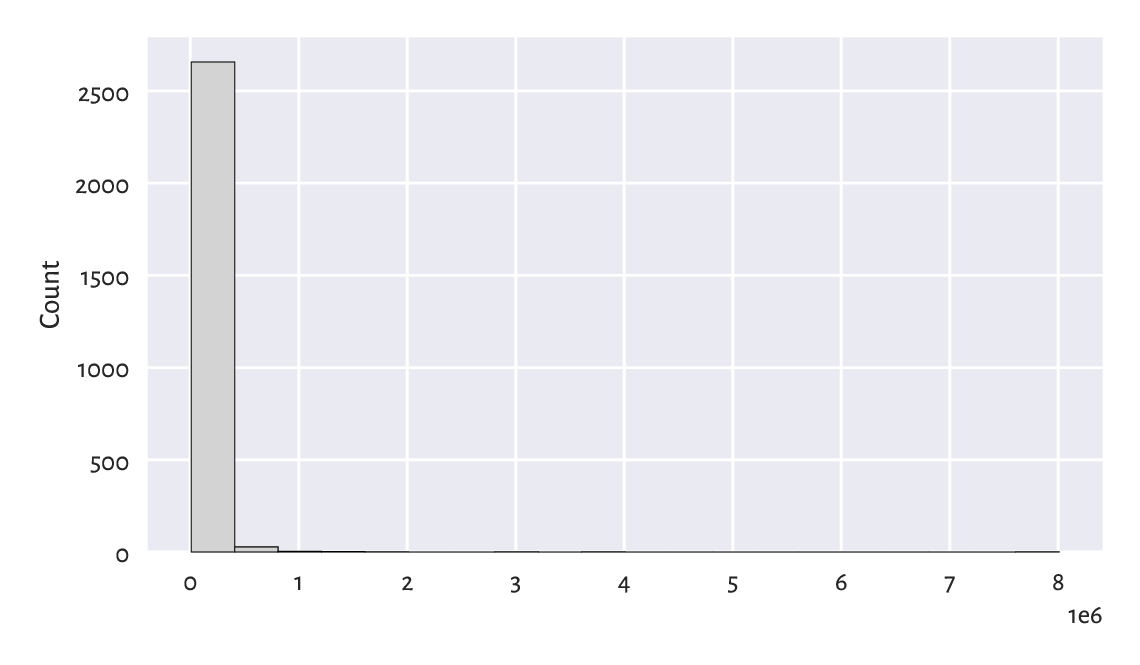Figure 4.8 Histogram of the large_cities dataset; it is extremely heavy-tailed

This is why we should rather draw such a distribution on the logarithmic scale, see Figure 4.9.

sns.histplot(large_cities, bins=20, log_scale=True, color="lightgray")
plt.show()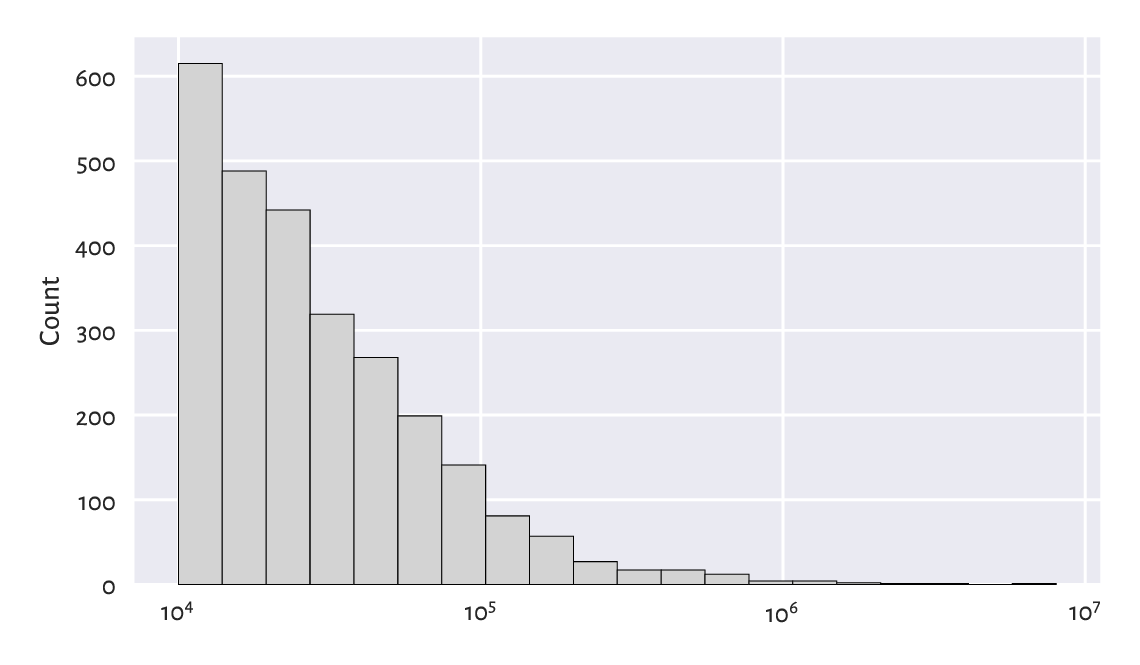Figure 4.9 Another histogram of the large_cities dataset; it is right-skewed even on a logarithmic scale

The log-scale on the x-axis does not increase linearly: it is not based on steps of equal sizes like 0, 1,000,000, 2,000,000, …, and so forth. Instead, now the increases are geometrical: 10,000, 100,000, 1,000,000, etc.

This is a right-skewed distribution even on the logarithmic scale. Many real-world datasets have a similar behaviour, for instance, the frequencies of occurrences of words in books. On a side note, Chapter 6 we will discuss the Pareto distribution family which yields similar histograms.

Exercise 4.9

Draw the histogram of income on the logarithmic scale. Does is resemble a bell-shaped distribution?

Exercise 4.10

(*) Use numpy.geomspace and numpy.histogram to apply logarithmic binning of the large_cities dataset manually, i.e., to create bins of equal lengths on the log-scale.

### 4.3.8. Cumulative Counts and the Empirical Cumulative Distribution Function

Let us go back to the heights dataset. The histogram in Figure 4.2 told us that, amongst others, 28.6% (1206 of 4221) of women are approximately 160.2±2.65 cm tall.

However, sometimes we might be more interested in cumulative counts, see Figure 4.10. They have a different interpretation: we can read that, e.g., 80% of all women are no more than ca. 166 cm tall (or that only 20% are taller than this height).

sns.histplot(heights, stat="percent", cumulative=True, color="lightgray")
plt.show()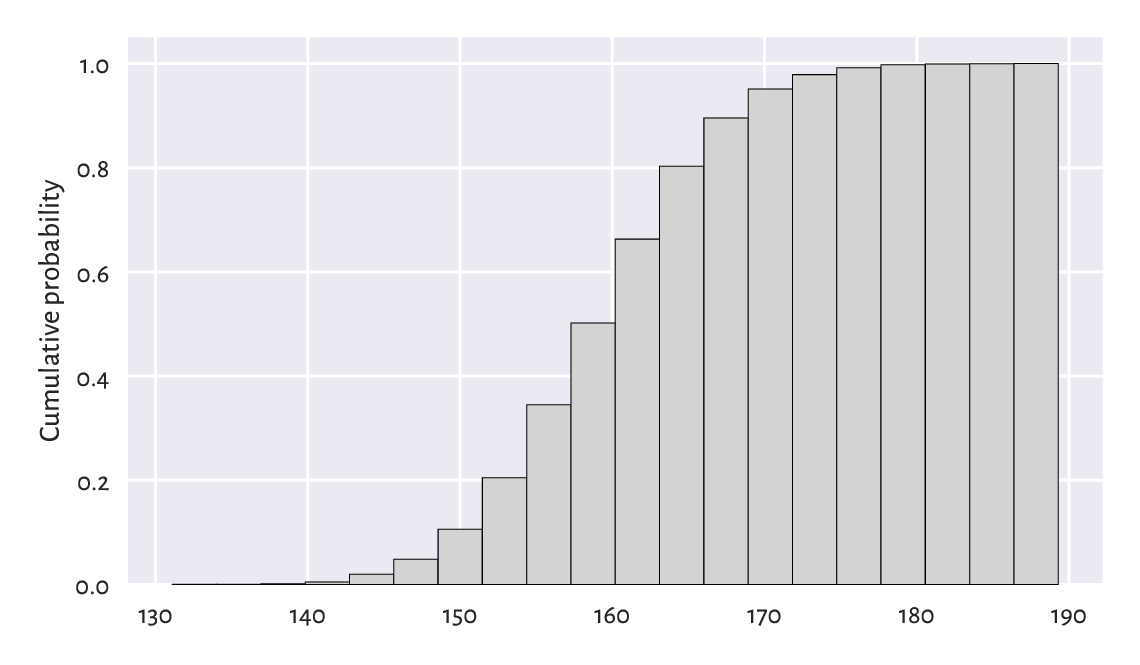Figure 4.10 Cumulative histogram of the heights dataset

Very similar is the plot of the empirical cumulative distribution function (ECDF), which for a sample $$\boldsymbol{x}=(x_1,\dots,x_n)$$ we denote as $$\hat{F}_n$$. And so, at any given point $$t\in\mathbb{R}$$, $$\hat{F}_n(t)$$ is a step function9 that gives the proportion of observations in our sample that are not greater than $$t$$:

$\hat{F}_n(t) = \frac{| i: x_i\le t |}{n}.$

We read $$| i: x_i\le t |$$ as the number of indexes like $$i$$ such that the corresponding $$x_i$$ is less than or equal to $$t$$. It can be shown that, given the ordered inputs, $$x_{(1)}\le x_{(2)}\le \dots\le x_{(n)}$$, it holds:

$\begin{split} \hat{F}_n(t) = \left\{ \begin{array}{ll} 0 & \text{for }t<x_{(1)}, \\ k/n & \text{for }x_{(k)} \le t<x_{(k+1)}, \\ 1 & \text{for }t\ge x_{(n)}. \\ \end{array} \right. \end{split}$

Let us underline the fact that drawing the ECDF does not involve binning – we only need to arrange the observations in an ascending order. Then, assuming that all observations are unique (there are no ties), the arithmetic progression $$1/n, 2/n, \dots, n/n$$ is plotted against them, see Figure 4.1110.

n = len(heights)
heights_sorted = np.sort(heights)
plt.plot(heights_sorted, np.arange(1, n+1)/n, drawstyle="steps-post")
plt.xlabel("$t$")  # LaTeX maths
plt.ylabel("$\\hat{F}_n(t)$, i.e., Prob(height $\\leq$ t)")
plt.show()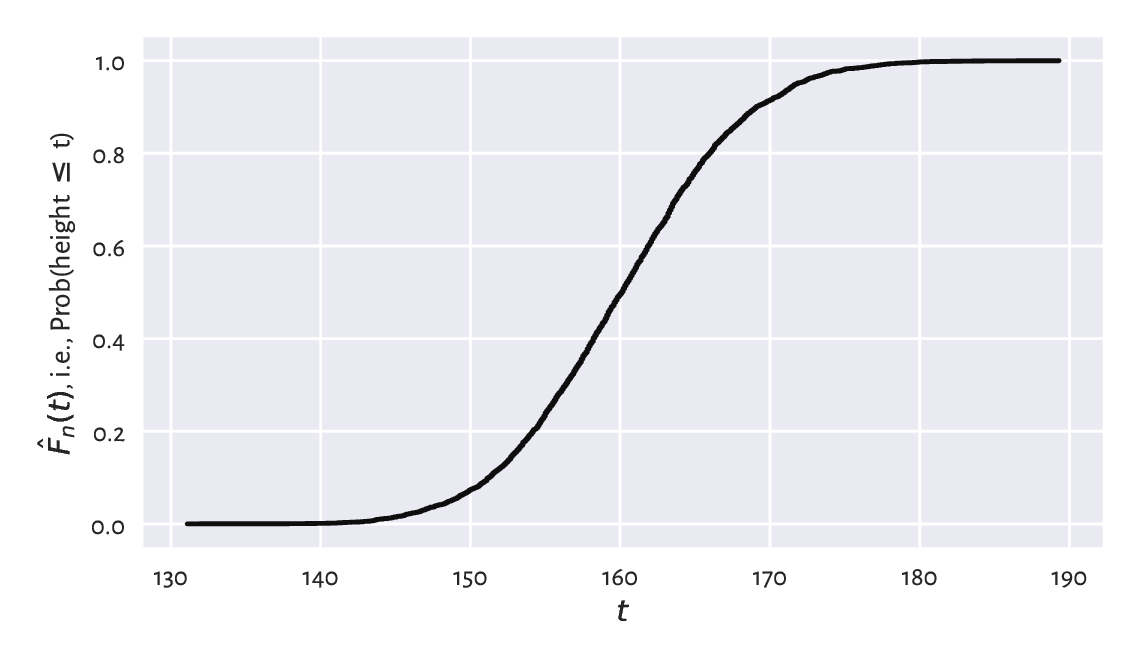Figure 4.11 Empirical cumulative distribution function for the heights dataset

Exercise 4.11

Check out seaborn.ecdfplot for a built-in method implementing the drawing of an ECDF.

Note

(*) Quantiles (which we introduce in Section 5.1.1.3) can be considered a generalised inverse of the ECDF.

## 4.4. Exercises

Exercise 4.12

What is the difference between numpy.arange and numpy.linspace?

Exercise 4.13

(*) What happens when we convert a logical vector to a numeric one? And what about when we convert a numeric vector to a logical one? We will discuss that later, but you might want to check it yourself now.

Exercise 4.14

Check what happens when we try to create a vector featuring a mix of logical, integer, and floating-point values.

Exercise 4.15

What is a bell-shaped distribution?

Exercise 4.16

What is a right-skewed distribution?

Exercise 4.17

What is a heavy-tailed distribution?

Exercise 4.18

What is a multi-modal distribution?

Exercise 4.19

(*) When does logarithmic binning make sense?

1

(*) Vectors are directly representable as simple arrays in the C programming language, in which numpy procedures are written. Hence, operations on vectors will be very fast provided that we are using the functions working on them as a whole. The readers who have some background in other lower-level languages, will need to get out of the habit of processing individual elements using a for-like loop.

2

DuckDuckGo also supports search bangs like “!numpy linspace” which redirect to the official documentation automatically.

3

When we write See also, it means that this is an exercise for the reader (Rule #3), in this case: to look something up in the official documentation.

4

If $$\boldsymbol{x}\in \mathbb{R}^n$$, then we often say that $$\boldsymbol{x}$$ is a sequence of $$n$$ numbers, a (numeric) $$n$$-tuple, a $$n$$-dimensional real vector, a point in a $$n$$-dimensional real space, or an element of a real $$n$$-space, etc. In many contexts, they are synonymic.

5

Some textbooks denote the i-th order statistic with $$x_{i:n}$$, but we will not.

6

A rather traditional name, but we will get used to it.

7

In fact, we have a proposition stating that the sum or average of many observations or otherwise simpler components of some more complex entity, assuming that they are independent and follow the same (any!) distribution with finite variance, is approximately normally distributed. This is called the Central Limit Theorem and it is a very strong mathematical result.

8

Gombrowicz, Nałkowska, Miłosz, Tuwim, etc. – I recommend.

9

We cannot see the steps in Figure 4.11, because the points are too plentiful.

10

(*) We are using (La)TeX maths typesetting within "$...$" to obtain nice plot labels, see [O+21] for a good introduction.